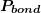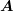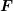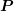### A Brief Guide to Engineering Financial Calculations: Present Worth Analysis

Future values are a set of monetary values expressed in terms of the time at which each of the transaction occurs. Each amount is expressed in the value of the currency at that particular time, referred to as future dollars or more generally as “as-spent” dollars.

Present values (PV) convert the set of future values to the equivalent set of present sums in terms of a single value of currency at a single point in time, referred to as “today’s dollars.” This conversion is done using (P|F,i,n), whereis the discount rate (or interest rate or return rate).

In Present Worth analysis, the focus is on the future. Any values from a time before the present time are ignored. These are called sunk costs.

The sum of the PV values over the course of the overall time determines the net present value (NPV) of financing plus the returns on the investment over and above the interest rate. A company usually has a discount rate that is the minimum that is acceptable as a “hurdle rate”: the Minimum Acceptable Rate of Return (MARR).

When MARR is used as the interest rate, then the NPV needs to be greater than zero to be accepted. (That’s why it is called a hurdle rate: the NPV has to be more than zero to clear the bar at MARR.) The Weighted Average Cost of Capital (WACC) is another hurdle rate that a company may use, which represents the cost of its financing and its overall level of investment risk. If the investment does not provide a return at that rate or better, then the company’s performance slips.

### Calculating the Present Market Value of a Bond

When assessing an investment, it is important to consider what its current market value, to determine whether it is still a worthwhile investment or it should be sold.

In the case of a bond, the investment has a fixed “coupon” rate, meaning that the same amount is paid at the end of each year for a specified number of years, as a percentage of the face value of the bond (the Principal). At the end of the final period, you also get the Principal back (the original amount).  The market interest rate is the discount rate that affects the future value of those amounts.

In reality, the market interest rate changes all the time, but for our purposes for future value calculation we assume that it is constant, because we have no information to make us believe that the discount rate is going to be different from what it is right now.

The market value of the bond now is simply the sum of the present valueof the uniform series of remaining annuity amountsplus the present value of the principal repayment(the original amount), using the market interest rate for the discount rate. The periodis based on how many remaining payments will be made; annuity amounts that have already been paid in the past are not counted. The principal repayment of the original amount at the end of the term is a future value, which is why we have to calculate its discounted present value for the investment decision being made today.

### Present Worth Analysis Using A Spreadsheet

Present Worth Analysis is most easily done using a spreadsheet program. The formulæ shown in the section above are useful for specific situations; but in reality, most cash flows are not as well behaved as the idealized series.

Calculation

To set up the spreadsheet, each future valueis listed with the time at which occurs, expressed as a number of time intervals(usually years). The interest rateis entered into a cell on its own. Usually, the interest period is one year, but not necessarily.

Recall that the present valueof a sum that isperiods in the future (the future value) is calculated by using a negative value for the number of compounding periods:

P = F x ( 1 +i )n

This formula is used to calculate the present value of each of the individual future values.

In the example spreadsheet, the expression that is typed into cell \$C\$5 to calculate the present valueof \$100 at the end of period 1:

P = 100 x ( 1 + 0.03 )-1  is

=B5*(1+\$B\$2)^(-A5).

Note that an absolute cell reference is used for the interest rate in cell \$B\$2.

The future values are entered in column B starting “today” in row 4, with the value one period later in row 5, etc.

The value in cell \$C\$6 calculates the present value at the end of year 2, which in this case is  P = 100 x ( 1 + 0.03 )-2, the formula in the cell is =B6*(1+\$B\$2)^(-A6). The value in cell \$C\$7 to calculate the present value at the end of year 3, or  P = 100 x ( 1 + 0.03 )-3, is =B7*(1+\$B\$2)^(-A7).

The NPV is simply the sum of the present values. In Excel, use the SUM( ) function. In this example the formula in cell \$C\$8 is

=SUM(C4:C7)

In this particular example, the NPV of the series happens to be the same as the Uniform Series Present Worth in the case of P =100 x (P/A, 3%, 3).

Here is a tip for setting up a spreadsheet for NPV: Use an absolute reference for the interest rate. Remember that the convention for an absolute reference to a cell is to use dollar signs in front of the column (letter) and/or the row (number), \$B\$2 in this example. In this way, the present value is calculated by pointing directly to the cell that has the number that you use repeatedly, in this case, the interest rate. A common error can occur when setting up the spreadsheet by copying cells into another location that end up referring to the wrong cell. Check your calculation formulæ in the cells to make sure you are using the correct parameters from other cells.

### Rate of Return

The internal rate of return (IRR) is the interest rate for which the sum of present values totals to zero. This is the rate of return at which the benefits of the investment are the same as its costs. If the IRR is greater than MARR, then it is a worthwhile investment.

If IRR is higher than MARR, then later future sums have more value at the MARR hurdle rate than at the IRR rate, which means that the investment will deliver value beyond what is demanded at the MARR.  If IRR is below MARR, then later future sums have less value at the MARR hurdle rate than at IRR rate, and so the investment will not deliver value required at MARR.

Calculation:

Tabulate a time series set of future values on a spreadsheet and calculate their individual present values using a first guess at the IRR interest rate. Calculate the Net Present Value of the series of Present Values. Then, use the Excel solver function to make this IRR Present Value total equal to zero by solving for the IRR interest rate, which is chosen by selecting the cell that contains the interest rate.

It is good practice to create an additional column in a spreadsheet for each present value case (e.g. MARR and IRR). You should question an IRR in the bazillions of percent; a common error is not putting the correct signs on costs or benefits.

Solving for i of a Cash Flow Series by Interpolation Using Interest Tables

We can find an approximate solution for the interest rate for a cash flow series which we can express as a formula, provided thatis the only unknown, by finding the value of the formula factor, and then interpolating in the interest table to find the approximate value of.

Incremental IRR

Incremental IRR (IIRR) is used for an investment that is being considered as an alternative to another investment. The investment with the lower capital cost is considered to be the base case. The cash flow of the higher-capital-cost alternative (in future dollars) is subtracted from the cash flow of the base investment (also in future dollars) to give the incremental cash flow. The IIRR is then found using an iterative solution (such as Solver in Excel) for the series of present values of the incremental cash flow that gives an NPV of \$0. If the IIRR is greater than MARR, then the higher capital cost alternative should be selected, because it adds enough extra value to be worthwhile. In fact, if the IIRR is greater than the IRR of the base investment, then the incremental investment adds more value to the investment on a relative basis than the base investment does.

### Other Analysis Techniques

Benefit-Cost Analysis

The Benefit-Cost ratio compares the Present Worth of the benefits of an investment PWbenefits, and the Present Worth of the costs PWcosts

BCR = PWbenefits / PWcosts

If BCR < 1, then the benefits are less than the costs, and so the investment is not worthwhile.

Incremental Benefit-Cost Analysis

Incremental Benefit-Cost analysis compares increasing costly alternatives.

• For each option, calculate the PW of its benefits the PW of its costs, and its Benefit to cost ratio. Reject any option that has a BCR < 1.
• Arrange the remaining options in ascending order of costs.
• Then calculate the incremental BCR between cases, starting with the lowest two cost options. As analysis proceeds, reject any option that gives an incremental BCRs that is less than 1, and use the next higher cost option to calculate the incremental BCR.
• Once all the valid incremental BCRs have been calculated, choose the option with the highest cost that still has an acceptable incremental BCR (that is, greater than 1). Note that the choice will not necessarily have the highest BCR.

Payback

Payback happens when an investment has paid for itself. The point at which the cumulative sum of values becomes positive is when payback occurs.

For engineering projects, the payback time is usually counted as the time from start-up (that is, the first period in which there is positive cash flow) to the point at which the sum of the series of time values goes positive, rather than counting from the beginning of the project itself. Payback usually happens in the middle of a period, but payback period is usually expressed as an integer: the end of that period minus beginning of start-up. For example, if an engineering project started at the beginning of year 1, started up sometime in year 4, and the sum of values went positive sometime in year 11, then the payback time is

11 – 4 = 7 years. Some people interpolate within a year to get more precision, but it’s not usually worth the extra effort, given the inherent uncertainties in such analyses.

Simple payback is found from the series of future values.

Discounted payback is found from the series of present values (the discounted values of the future sums).

Break-Even Analysis

Break-even analysis considers the effect of a parameter in an investment option on its equivalence to another investment option. The key is to express one investment option in terms of the variable to be solved and the equivalent value of the other option.

If the cash flow is simple, then it is often easiest to solve for a parameter directly using an interest formula and solving by interpolation from the interest tables. Of course, a spreadsheet can be used with the Solver function to find the value of the parameter.

Solving for n of a Cash Flow Series by Interpolation Using Interest Tables

Break-even analysis is often concerned with the time period associated with different investment options. Interpolation can also be used to find the value of. In Lecture 27, there is a break-even analysis problem that determines how many years a corrosion-resistant part has to last to have the equivalent uniform annual cost of the untreated part. The untreated part costs \$350 and lasts 6 years, so the uniform annual costs is \$350 x (A|P,10%,6) = \$80.36. The corrosion-resistant part equivalent uniform annual cost (Option B) must be at least this low, so we have to find the number of years that will give the same amount, i.e., \$500 (A|P,10%, n) = \$80.36. So now we know that (A|P,10%, n) = \$80.36 / \$500 = 0.1607, and we look in the Uniform Series Capital Recovery Table (on slide 13 of Lecture 21) and find that in the column for (A|P,10%,n) the value 0.1627 for= 10 and 0.1540 for=11. That means that the solution lies somewhere between 10 and 11 years. By interpolation, we can solve= 10 + (0.1627 – 0.1607)/(0.1607 b- 0.1540) = 10.23 years.

Picture

What Is Included in the Cash Flow Series for an Investment Project

In evaluating an investment, only future costs are considered. The cash flow series should only include the incremental costs to do the project, and none of the costs associated with running the current business. For example, allocation of costs for head office overheads does not enter into a cash flow analysis, since no incremental money is spent. (The head office would run whether the project went ahead or not.) Providing an accounting reserve for future expenditures is a common accounting practice (recall that allowances are used to blend out expenditures); but cash flow forecasts use actual cash flows that are expected to occur, not allowances. Some projects may do preliminary project development work as part of regular business activities. These sunk costs would not be attributed to the project.